# Algebra II : Setting Up Inequalities

## Example Questions

1 2 4 Next →

### Example Question #31 : Setting Up Inequalities

Twice a number,, is less than twice the quantity ofsubtracted from 4. Which of the following inequalities represents this statement?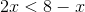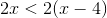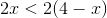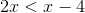Explanation:

In order to set up the equation for this inequality, break down the sentence into parts.

Twice a numberIs less than: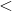The difference of four and the number x :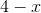Twice the difference of four and the number: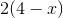Combine all the terms and signs.

The answer is:.

1 2 4 Next →

### All Algebra II Resources